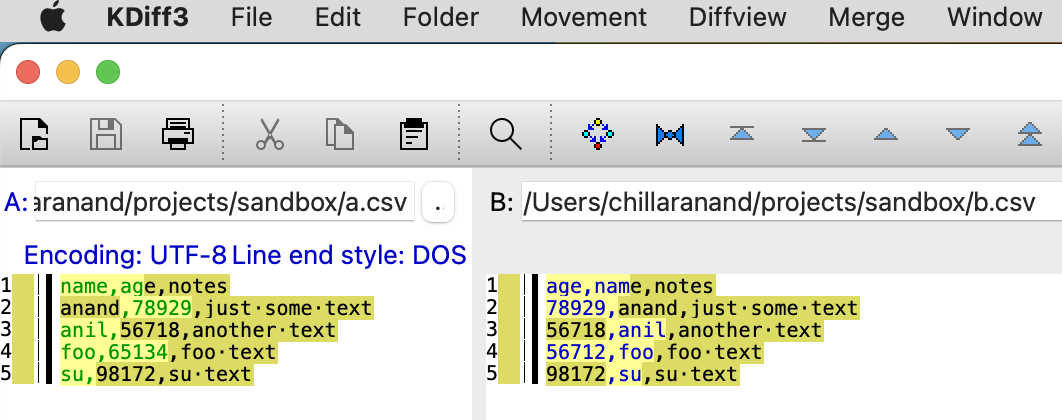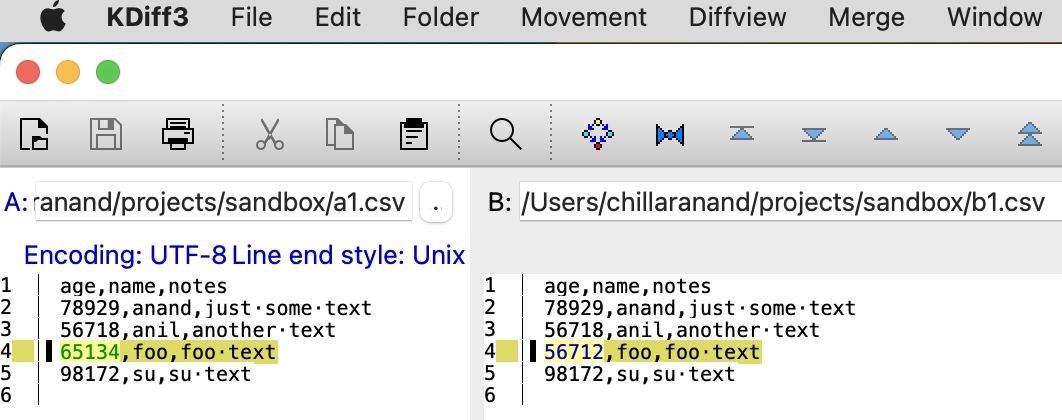# Rearrange CSV columns alphabetically from CLI

We can use tools like KDiff3 to compare two CSV files. But, it is difficult to identify the diff when the columns are not in the same order.

For example, look at the below output of 2 simple csv files.Even though it highlights the diff, it is difficult to identify the diff because the columns are not in the same order. Here is the same diff after rearranging the columns alphabetically.Now, it is easy to identify the diff.

#### Rearrange CSV columns alphabetically

We can write a simple python script using Pandas1 as follows.

```#! /usr/bin/env python3

"""
re-arrange columns in alphabetical order
"""
import sys

import pandas as pd

def colsort(df):
cols = list(df.columns)
cols.sort()
return df[cols]

def main():
input_file = sys.argv
try:
output_file = sys.argv
except IndexError:
output_file = input_file
df = colsort(df)
df.to_csv(output_file, index=False)

if __name__ == '__main__':
main()
```

We can use this script as follows.

```\$ python3 rearrange_csv_columns.py input.csv output.csv
```

Instead of writing a script by ourselves, we can use `miller`2 tool. Miller can perform various operations on CSV files. We can use `sort-within-records` to sort the columns.

```\$ mlr --csv sort-within-records -f input.csv > output.csv
```

#### Conclusion

We can use `miller` to sort the columns in a CSV file. This will help us to identify the diff easily when comparing two CSV files.